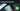# Hackerrank Solution of Compare the Triplets in Golang

August 25th, 2018
|

Solution for hackerrank warmup question compare the triplets in golang. In this question we will use if-else statement and arrays in golang for the first time along with for loops that we covered earlier.

# Problem Statement

The question can be found at this link. The problem statement states that we need to compare two sets of inputs and provide a result based on the comparison.
Couple of things to note here is

• We need to take six inputs, 3 on each line
• we are solving this in golang (assuming we understand basic for loops, input, output in golang, refer to my earlier posts in case you need a refresher )

# Challenges

• Choose our preferred language as golang on hackerrank. The moment we do that, we get some 50-60 lines of code which are very unfamiliar to someone who is new to language.
• To solve this problem only thing we need to know is how to take input, show output, iterate over an array, use if-else statement and arrays in golang

# Solution

This is the template you get on hackerrank for this problem statement.

``````package main

import (
"bufio"
"fmt"
"io"
"os"
"strconv"
"strings"
)

// Complete the compareTriplets function below.
func compareTriplets(a []int32, b []int32) []int32 {

}

func main() {

stdout, err := os.Create(os.Getenv("OUTPUT_PATH"))
checkError(err)

defer stdout.Close()

writer := bufio.NewWriterSize(stdout, 16 * 1024 * 1024)

var a []int32

for i := 0; i < 3; i++ {
aItemTemp, err := strconv.ParseInt(aTemp[i], 10, 64)
checkError(err)
aItem := int32(aItemTemp)
a = append(a, aItem)
}

var b []int32

for i := 0; i < 3; i++ {
bItemTemp, err := strconv.ParseInt(bTemp[i], 10, 64)
checkError(err)
bItem := int32(bItemTemp)
b = append(b, bItem)
}

result := compareTriplets(a, b)

for i, resultItem := range result {
fmt.Fprintf(writer, "%d", resultItem)

if i != len(result) - 1 {
fmt.Fprintf(writer, " ")
}
}

fmt.Fprintf(writer, "\n")

writer.Flush()
}

if err == io.EOF {
return ""
}

return strings.TrimRight(string(str), "\r\n")
}

func checkError(err error) {
if err != nil {
panic(err)
}
}``````
• First go ahead and delete everything in the pre-existing template.
• Now as usual, create our outer program structure. This is not our final snippet, we will create a much optimized template in coming posts for fast I/O, but for now just go ahead and create a simple structure that we have been using this far.
``````package main
import "fmt"

func main(){

}``````

If this seems unfamiliar, please refer to my earlier posts. Next thing we need is to take 6 inputs. There are two options :-

• Take six variables
• Use arrays

Lets learn arrays!!!. So now I will declare 2 arrays of length 3 each and take inputs in those arrays.

``````    var a int
var b int``````

This is the syntax for declaring an array in golang. Like variable, type comes later and we provide the length of array in `[]`. Length of an array in golang is fixed. Since both a, b are of same type, we could have clubbed the declaration like we club variable declaration. Now we need to take our inputs, so ahead and add these lines of code.

``````fmt.Scan(&a,&a,&a)
fmt.Scan(&b,&b,&b)``````

Nothing unusual, referencing indexes 0,1,2 and taking inputs. Now we need to declare two more variables for storing result of the comparison, loop over the values and compare them.

``````    var ar,br int
for i:=0;i<3;i++{
//compare here
}``````

We are looping three times, as that's the length of our arrays. Next we need to add our comparison logic in there and print the result.

``````        if a[i] > b[i] {
ar++
}else if b[i] > a[i] {
br++
}
//after for
fmt.Println(ar,br)``````

# If-ELSE in Golang

If-else in golang is also similar to for, `()` not mandatory , `{}` is must. rest is pretty much self explanatory. Notice the use of else if insted of else, we need to take care of case where `a[i]=b[i]` no value should be incremented there. That is the reason for using else if instead of just an else.

Now your complete code should look like

``````package main
import "fmt"

func main(){
var a int
var b int
fmt.Scan(&a,&a,&a)
fmt.Scan(&b,&b,&b)
var ar,br int
for i:=0;i<3;i++{
//compare here
if a[i] > b[i] {
ar++
}else if b[i] > a[i] {
br++
}
}
fmt.Println(ar,br)
}``````